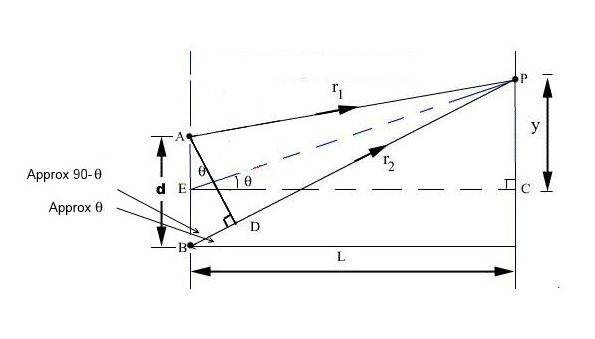# How we know that these angles are equal ?

Samia qureshi
Member advised to use the homework template for posts in the homework sections of PF.
https://fbcdn-sphotos-a-a.akamaihd.net/hphotos-ak-xpa1/v/t1.0-9/13529050_1033411326734648_2364124398862717743_n.jpg?oh=991ce9f095c712dd7789ce0819be581e&oe=58016FFA&__gda__=1475707536_89e5606d703064d4c1ccd2e87d665c1b

are these alternate interior angle or exterior angle? is it just because of mathematical rule of geometry or what makes these angle equal?

## Answers and Replies

Homework Helper
They are not the same ... the diagram is showing you an approximation.

If L >> d, then the rays AP EP and BP are almost sort of parallel. Now do the geometry.

•Delta2, Nidum, Samia qureshi and 1 other person
Homework Helper
Gold Member
I was making a version of your diagram when Simon beat me to it..•Nidum and Samia qureshi
Samia qureshi
Thank youGold Member
I'm always suspicious when I see a woolly question about geometry where the lines on supplied diagram are all labelled but there is no reference to them in the question .

Could it be that the actual question is whether there is any possible relationship between the lengths of the labelled lines which makes the problem angles equal ?

There is certainly one such relationship possible where EP and BP are the same length ie where EP coincides with BP .

For a given angle and one given length the other line lengths could now be determined .

Homework Helper
Gold Member
Could it be that the actual question is
Simon's interpretation seems rather more likely.

Homework Helper
Gold Member
It's certainly recognisable as a drawing associated with diffraction gratings/interference.

Vibhor
Simon's interpretation seems rather more likely.
When L >> d, then AP EP and BP are parallel. What is the reasoning behind this result ? Can it be proven mathematically that the three lines are almost parallel under the approximation L >> d ?

Homework Helper
Gold Member
When L >> d, then AP EP and BP are parallel. What is the reasoning behind this result ? Can it be proven mathematically that the three lines are almost parallel under the approximation L >> d ?
Say the lines have started at A and B and they meet each other at P. L is the distance from the starting point at which they meet. If distance between the lines d is negligible compared to L, they can be treated as two parallel lines. For two actual parallel lines, the distance between them is negligible compared to the distance at which they meet, which is infinity. Hence, their slopes are equal. So, here, the two lines with very small d(in mm) and very large L (in meters) can be approximated as two parallel lines. Their slopes will be almost equal.

Last edited:
Vibhor
If distance between the lines d is negligible compared to L, they can be treated as two parallel lines.
Why ? This is what I asked in the previous post. Could you prove it mathematically ?

Homework Helper
Gold Member
Why ? This is what I asked in the previous post. Could you prove it mathematically ?
For the lines with d<<L, the meeting distance L is very high compared to their separation d. For actual parallel lines, the meeting distance is infinity which means it is very very large compared to their separation. So, the lines with d<<L can be practically treated as parallel lines.
Mathematically speaking, their slopes are almost equal. Draw a horizontal line from A and let it cut the screen at point M. If you calculate the slopes of AP and BP, you'll get slope of AP=PM/AM
and slope of BP=(PM+d)/AM. Let's say AM=1m, PM=1m and d=1mm. So, PM≈PM+d. If PM is small, say 10mm, PM/AM≈(PM+d)/AM since AM is in meters and PM and d are in a few mm i.e. 10mm/1m≈11mm/1m. In both the cases, the slopes are almost equal.

Last edited:
•Vibhor
Homework Helper
Gold Member
Why ? This is what I asked in the previous post. Could you prove it mathematically ?
From the diagram in the opening post, the gradient of the lines AP and BP are
$$g_{AP}=\frac{y-d/2}{L} = \frac{y}{L}-\frac{d}{2L}$$
$$g_{BP}=\frac{y+d/2}{L}=\frac{y}{L}+\frac{d}{2L}$$
In the limit ##d\ll L##, the second term in each expression for the gradient goes to zero, and we see that the two gradients are equal. Therefore, the lines are parallel.

•cnh1995 and Vibhor
Vibhor
From the diagram in the opening post, the gradient of the lines AP and BP are
$$g_{AP}=\frac{y-d/2}{L} = \frac{y}{L}-\frac{d}{2L}$$
$$g_{BP}=\frac{y+d/2}{L}=\frac{y}{L}+\frac{d}{2L}$$
In the limit ##d\ll L##, the second term in each expression for the gradient goes to zero, and we see that the two gradients are equal. Therefore, the lines are parallel.

Very Nice !

Thanks